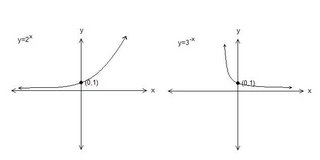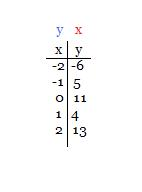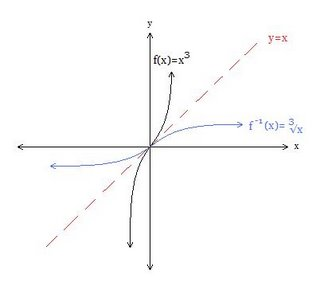## September 19, 2006

### Scribe Post: Day 8

Hey kids, it's Loso and I was today's class scribe. Today we were given three questions to do, to follow up on what we learned yesterday [technically speaking, it's what you all learned yesterday because I wasn't in class yesterday, SORRY!].

QUESTIONS:

1. Dave invests \$100 at 8% interest per year. How much does Dave have after 6 years if the interest is compounded;

a) annually
b) quarterly
c) continuously

2. The population of New Hampshire was 1 million in 1990. It doubles every 25 years. What is it today?

3. Describe all the features and properties of the graphs of:

a) y-2x
b) y=3-x

SOLUTIONS:

1. a) Since we're given a sufficient amount of information, we can use the A=P(1+r/n)tn formula. I'm pretty sure you all know how to plug in the information, but just for every one's sake, let's make sure.

A is what we're looking for.
Our principle amount is \$100 [P].
1 is taking 100% of what we already have and then adding it with the rate of interest.
Our rate of interest is 8%, and is 0.08 when written as a decimal [r].
6 is the amount of time we're looking at. [t]
n is the number of times we compound a year. In this case, we're compounding once every year.

A=P(1+r/n)tn
A=100[1+(0.08/1)](6)(1)
A=100(1.08)6

We were able to leave it at that for today.

b) We use the same formula, with the exception that we're compounding 4 times a year, instead of once a year.

A=P(1+r/n)tn
A=100[1+(0.08/4)](6)(4)
A=100(1.02)24

c)For c, we use A=Pert

A=Pert
A=100e(0.08)(6)
A=100e(0.48)

2. For this question, we use A=Ao(M)t/p

A=Ao(M)t/p
A=1,000,000(2)15/25

Umm, I kind of missed the answer. He was going through it pretty quickly. Hopefully I did that right.

3.These are what the graphs look like.

y=2x

-increasing function (or grows exponentially)
-asymptote is y=0
-DOMAIN (-∞, ∞)
-RANGE (0, ∞)
-y int. y=1
-no roots
-concaves up

y=3-x

-decreasing function (or exponential decay)
-asymptote is y=0
-DOMAIN (-∞, ∞)
-RANGE (0, ∞)
-y int. y=1
-no roots
-concaves up

Everything in purple is what is similar between both functions.

We then discussed how to describe what an inverse was.

VERBALLY: An inverse of a function is a function that undoes what the parent function does. You can also say, the inputs become outputs, and the outputs become inputs

He brought up the baby play and parent clean up example. I think we're all pretty clear of what the point of that was.

NUMERICALLY: This was the example we were given, basically showing how the y values become x values, and the x values become y values.GRAPHICALLY:Yeah, I know the lines look wrong, but it's the best I can do right now so bear with me. Near the end of the class, there was some discussion about one to one functions, which at this time is quite vague to me. It's late and my brain needs rest. Our homework is exercise 1.6, all odd questions including 10,18,28 and 30.

For the next scribe, I choose Suzanne. Have fun with that. =)